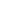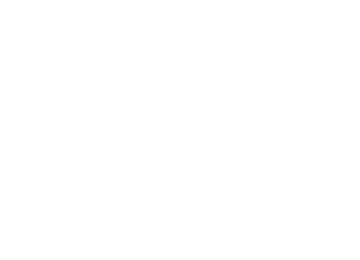###### Held on Jul 4, 2021
8:30 AM###### 54 Q’s · 180 marks
180 mins###### Languages
English#### Syllabus

##### Mathematics
• Circles
• Compound Angle
• Differentiations
• Limits & Continuity
• Relation and function
• Logarithm & its Properties
• Inverse Trigonometric Functions
• Trigonometric Identities & Equations
• Binomial Theorem
• Permutations & Combinations
• Straight Lines
• Functions & Graphs

##### Chemistry
• Coordination Compounds
• General Topics & Mole Concept
• Nuclear Chemistry
• Alkanes & Alkyl Halides
• Atomic Structure
• Chemical Bonding
• Energetics & Thermodynamics

##### Physics
• Geometrical Optics
• Work, Energy & Power
• Electrostatics
• Wave Optics
• Laws of Motion & Friction
• Surface Tension & Energy
• Centre of Mass & Linear Momentum
• Solids
• Viscosity & Terminal Velocity
• Simple Harmonic Motion
• Lenses